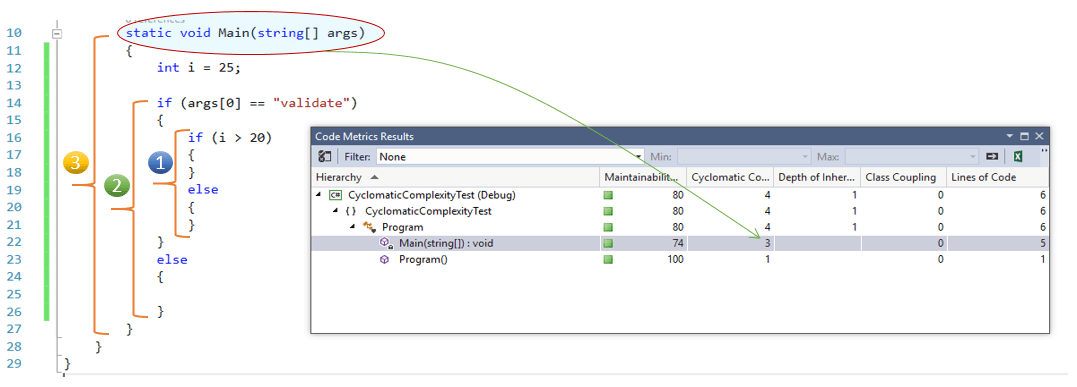# Understand the complexity and maintainability of your code using Code Metrics in Visual Studio – Cyclomatic Complexity

By | December 23, 2015This is the 5th post in this series on understanding code complexity and maintainability. In this post we are going to learn about Cyclomatic Complexity; which is an important aspect of the code metrics.  While other three metrics we discussed earlier – Line Of Code , Class Coupling and Depth of Inheritance define each and individual definition for the code metrics, the Cyclomatic complexity metrics defines the complexity within the code or logic. or in short it defines the “how complicated the structure of the code is”.

Cyclomatic complexity helps us by measuring the code complexity.  Higher the code complexity is,  the more code is complex.  The number of  the Cyclomatic complexity depends on how many different execution paths or control flow of your code can execute depending on various inputs.

The more the code paths, the more the code complexityUnderstanding Cyclomatic Complexity in Visual Studio

Let’s start understanding how the cyclomatic complexity number get calculate by creating a new console application; very similar to what we did for earlier post..

```class Program
{
static void Main(string[] args)
{
}
}
```

Then Run the Code Metrics tools from the project context menu. At the start you will see the code cyclomatic complexity is 2. Any class start with a complexity of 1, as we have an method added, the complexity increases to 2.Now start adding some logic with a basic if conditional statement as shown below..

```class Program
{
static void Main(string[] args)
{
int i = 25;
if (i > 20)
{
}
else
{
}
}
}
```

Run the Code Metric Tools, you will find the complexity for the Main() method is getting increased, eventually it is increasing the complexity for overall class and the project.Add an additional condition to the same code and run the code metrics tools once again

```class Program
{
static void Main(string[] args)
{
int i = 25;
if (args == "validate")
{
if (i > 20)
{
}
else
{
}
}
else
{
}
}
}
```

Below illustration for the code metrics is self explanatory, where you can see how each level of adding additional code complexity.Similar to this, if you add  more code blocks by adding any conditional statements or loops, it will slowly increase the number. and beyond some point of time, the code would be hard to understand.

The lower the number is, the less complex is your code. Cyclomatic complexity exceeding 20-25  (may vary ) is not recommended ; incase you see your code complexity exceeding this number make sure you are refactoring the code.

Here is the list of previous post from this series.Author: Abhijit Jana

Abhijit runs the Daily .NET Tips. He started this site with a vision to have a single knowledge base of .NET tips and tricks and share post that can quickly help any developers . He is a Former Microsoft ASP.NET MVP, CodeProject MVP, Mentor, Speaker, Author, Technology Evangelist and presently working as a .NET Consultant. He blogs at http://abhijitjana.net , you can follow him @AbhijitJana . He is the author of book Kinect for Windows SDK Programming Guide.